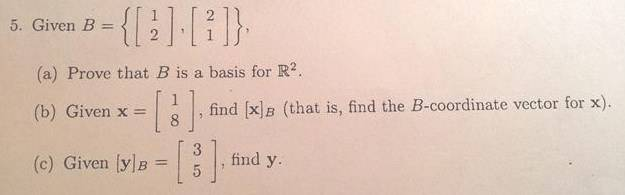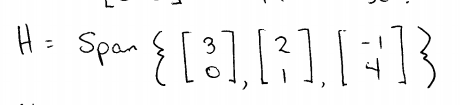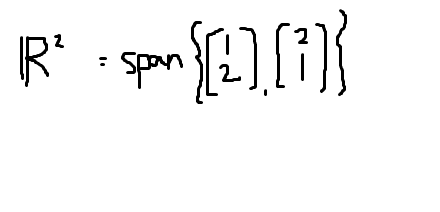# Trouble understanding spans of vector spaces

Hey guys

So the problem I'm having here is spans!

I know that a basis of a vector space is a linearly independent spanning set

So the linearly independent part is pretty easy...looking at whether or not vectors are linear combinations of the others (right? Or do I have to look at it by inspection and see whether or not one is a linear combination of the other? Or is that what I'm doing essentially? I'm pretty lost!), but how do I tell if a set of vectors is a span or not?

Like here's a question that I sort of understand but I don't get how to show if it's a span or not. **note this is not a homework question...it's a question on my practice test**So I turn these two vectors into a matrix A, and do a row reduction to see that it does have 2 pivot positions and is indeed linearly independent, but how do I know whether or not it's a spanning set?

Does it have something to do with the funny brackets that they write?

Another thing I see is them writing stuff like this:I mean what's the difference between writing span in front and not writing it? One is a span and the other is just a set of vectors? Blehhh :(

Edit: I think I'm sorta starting to understand it...so basically if I write this it makes sense, correct? (for the first question):Because of this: H = span{b1, b2, ... , bp} where B = {b1, b2, ... , bp}?

Basically the span of the basis is equal to its parent vector space?

Thanks,
Elliott

Last edited:

Hey guys

So the problem I'm having here is spans!

I know that a span of a vector space is a linearly independent spanning set

A basis is a linearly independent spanning set. Is that what you meant to say?

A spanning set "covers" the entire vector space by means of linear combinations. That is, any vector can be expressed as a linear combo of the vectors in the spanning set.

A basis is a linearly independent spanning set. So it's a spanning set, but a minimal one -- if you threw out any member of a basis, the resulting set would not span.

Does that help to clarify?

So the linearly independent part is pretty easy...looking at whether or not vectors are linear combinations of the others (right? Or do I have to look at it by inspection and see whether or not one is a linear combination of the other? Or is that what I'm doing essentially? I'm pretty lost!), but how do I tell if a set of vectors is a span or not?

By inspection, or some particular technique if they've gotten to that in the book yet. Main point is to understand the concept. If one of the vectors in the spanning set can be written as a linear combo of the others, then the spanning set's not a basis -- because you could throw out the extra vector and not lose any information, if you think of it that way.

Sorry, I didn't look at the attachment. But I think if you get the terminology and concepts clear, it helps a lot with the specific techniques.

A basis is a linearly independent spanning set. Is that what you meant to say?

A spanning set "covers" the entire vector space by means of linear combinations. That is, any vector can be expressed as a linear combo of the vectors in the spanning set.

A basis is a linearly independent spanning set. So it's a spanning set, but a minimal one -- if you threw out any member of a basis, the resulting set would not span.

Does that help to clarify?

Yea sorry that's what I meant to say! Basis not span!

By inspection, or some particular technique if they've gotten to that in the book yet. Main point is to understand the concept. If one of the vectors in the spanning set can be written as a linear combo of the others, then the spanning set's not a basis -- because you could throw out the extra vector and not lose any information, if you think of it that way.

Sorry, I didn't look at the attachment. But I think if you get the terminology and concepts clear, it helps a lot with the specific techniques.

Yes what you said did help but I still do not understand how to know whether or not a set of vectors given is a span or not. Like saying a basis is a 'linearly independent spanning set'. How do you know the spanning set part?

mathwonk
Homework Helper
it takes at least n vectors to span an n dimensional space.

a one diml space is spanned by any set that contains at least one non zero vector.

a 2 diml space is spanned by any set containing at least 2 non collinear vectors.

a 3 diml space is spanned by any set containing at least 3 non coplanar vectors.

get the idea?

Deveno
there some different ways to show spanning.

one way is by arguing by dimensions (how many n-dimensional subspaces does an n-dimensional vector space have?).

another way is to prove it directly: if your basis is {b1,...,bn}

demonstrating that for any given v in your vector space, you can actually produce the coefficients of the basis vectors that make a linear combination of the basis add to v.

for example, in R2, an arbitrary vector is (x,y).

if we have some vectors (u1,u2) and (v1,v2) that we suspect might span R2, then we should be able to find a and b such that:

a(u1,u2) + b(v1,v2) = (x,y).

in other words, some formula for a and b that only depends on x and y.

(note: the u's and the v's will be actual real numbers, not variables. i just didn't explicit say which numbers, because the mods are mad enough at me as it is).

HallsofIvy
Homework Helper
To show that set A spans vector space V, show that any vector in V is a linear combination of the vectors in set A.

For example, in your posted problem, you are given that
$$E=\left\{\begin{bmatrix}1 \\ 2\end{bmatrix}, \begin{bmatrix}2 \\ 1\end{bmatrix}\right\}$$
and are asked to show that it is a basis for $R^2$. That means that you must show that it spans $R^2$ and that the vector are independent.

To show that it spans $R^2$:
Any vector in $R^2$ is of the form
$$\begin{bmatrix}x \\ y\end{bmatrix}$$
for x and y real numbers. You need to show that there exist real number, a, b, such that
$$a\begin{bmatrix}1 \\ 2\end{bmatrix}+ b\begin{bmatrix}2 \\ 1\end{bmatrix}= \begin{bmatrix}a+ 2b \\ 2a+ b\end{bmatrix}= \begin{bmatrix}x \\ y\end{bmatrix}$$
That matrix equation is equivalent to the two equations a+ 2b= x and 2a+ b= y. Show that there exist such a and b by actually solving the two equations for a and b, in terms of x and y. (If you multiply the second equation by 2 and subtract from the first, you eliminate b and can solve for a. If you multiply the first equation by 2 and subtract from the second, you eliminate a and can solve for b.)

Show that they are independent by showing that
$$a\begin{bmatrix}1 \\ 2\end{bmatrix}+ b\begin{bmatrix}1 \\ 2 \end{bmatrix}= \begin{bmatrix}a+ 2b \\ 2a+ b\end{bmatrix}= \begin{bmatrix}0 \\ 0\end{bmatrix}$$
has only a= b= 0 as solutions. Again, that reduces to a+ 2b= 0 and 2a+ b= 0. Solve those two equations.

Basically, a set of vectors is a "basis" for a vector space if and only if every vector in the vector space can be written as a linear combination of the vectors in the given set in only one way. The fact that every vector can be written as a linear combination says that the set "spans" the space. The fact that there is only one such combination says that they are "independent".